#1

## 請問 Excel 有方法產生一千萬個介於0~1之間的不重複亂數嗎？

=IF(COUNTIF(\$A\$1:\$J\$1000000,A1)>1,RANDBETWEEN(0,10000000)/10000000,A1) ，填滿工作表欄位 A1:J1000000，共一千萬個儲存格範圍。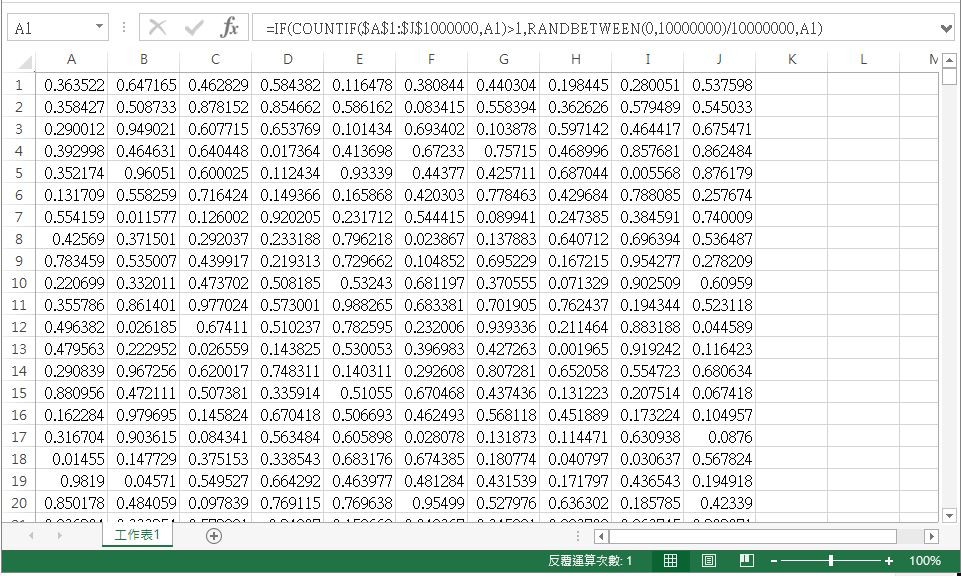1,048,576 列乘以 16,384 欄，所以您有換行嗎？

2013版本是過渡期品，所以很多公司都是2010直接跳2016，建議你拿最新版本的Excel2019測試，效率應該會提升很多。另外硬體效能也很吃緊，一般爛電腦開個10Mb的Excel就掛了，更別提你跑了12個多小時沒結果。

12個小時還好耶，我用C#加EPPLUS輸出XLSX，跑10000組號碼(1000列)都要花掉30秒，現在程式還在跑，有結果再貼上回文
froce iT邦大師 1 級 ‧ 2019-07-03 10:48:42 檢舉
C＃速度這麼慢？10000000組預估500分鐘！？

### 5 個回答

4

Excel我猜可能沒辦法太快,

dragonH iT邦超人 5 級 ‧ 2019-07-02 17:27:20 檢舉dragonH Excel是還沒崩潰，但鼠標就是一直轉圈圈超過12小時，轉到都快吐了還沒個結果...

1千萬筆本來就不小,

``````Private Sub test()
Dim mybag As New Collection ' 宣告一個放球的袋子，類型是Collection集合

totalRow = 9 '列數
totalNum = 72 '總數
For i = 1 To totalNum
mybag.Add (i / (totalNum + 1)) ' 依序放入1到49的號碼球
Next

For i = 1 To totalNum
R = Int(mybag.count * Rnd()) + 1 ' R 就是包包裡的球數*0~1之間的隨機數，集合跟陣列一樣，元素從1開始
Row = ((i - 1) Mod totalRow) + 1
Column = Int((i - 1) / totalRow) + 1
Cells(Row, Column).Value = mybag.Item(R) ' 按c值在相對的列上填入球號
mybag.Remove (R) ' 抽到的球就不要放回袋子(集合)裡了，直接拿掉
Next

End Sub
``````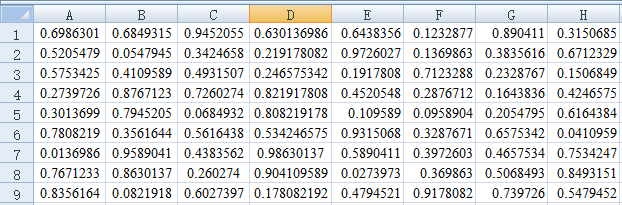1千萬筆你就自己慢慢跑,``````Private Sub test()
Dim mybag As New Collection ' 宣告一個放球的袋子，類型是Collection集合

totalRow = 1000000 '列數
totalNum = 10000000 '總數
For i = 1 To totalNum
mybag.Add (i / (totalNum + 1)) ' 依序放入（1到10000000的號碼球/號碼+1）
Next

For i = 1 To totalNum
R = Int(mybag.count * Rnd()) + 1 ' R 就是包包裡的球數*0~1之間的隨機數，集合跟陣列一樣，元素從1開始
Row = ((i - 1) Mod totalRow) + 1
Column = Int((i - 1) / totalRow) + 1
Cells(Row, Column).Value = mybag.Item(R) ' 按c值在相對的列上填入球號
mybag.Remove (R) ' 抽到的球就不要放回袋子(集合)裡了，直接拿掉
Next

End Sub
``````

VBA其實不會更快，很有可能更慢，頂多平手。

0

10,000,000 = 250 x 40,000
A..IP = 250 Column
1..40000 = 40000 Row

A1..IP40000

1~100，抽4個幸運兒。
1~25抽一位，
26~50、51~75、76~100，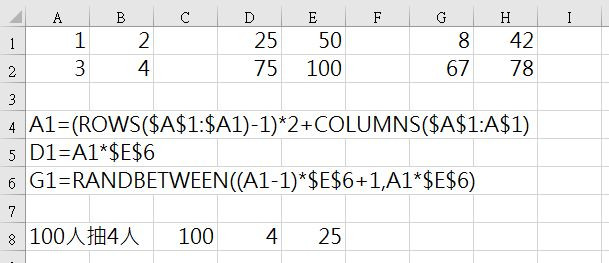2

golang，應該不用10分鐘吧:

``````package main

import (
. "fmt"
"math/rand"
"os"
"path"
"path/filepath"
"strconv"
"time"

"github.com/360EntSecGroup-Skylar/excelize"
)

func main() {
ex, err := os.Executable()
if err != nil {
panic(err)
}

exPath := filepath.Dir(ex)
book := path.Join(exPath, "Book1.xlsx")
f := excelize.NewFile()
f.SetActiveSheet(1)
columns := string{
"A",
"B",
"C",
"D",
"E",
"F",
"G",
"H",
"I",
"J"}

rand.Seed(time.Now().UnixNano())
p := rand.Perm(10000000)

for i := 1; i <= 1000000; i++ {
_p := p[i*10-10 : i*10]
for index, elm := range columns {
cell := elm + strconv.Itoa(i)
value := float64(_p[index]) / float64(10000000)
Println(cell, value)
f.SetCellValue("Sheet1", cell, value)

}
}
Println(book)
err = f.SaveAs(book)
if err != nil {
Println(err)
}

}

``````

https://drive.google.com/file/d/1iPOh_HqrH3Ysu2NzqE_1fwOhsDScg-wu/view?usp=sharing

froce感謝回答！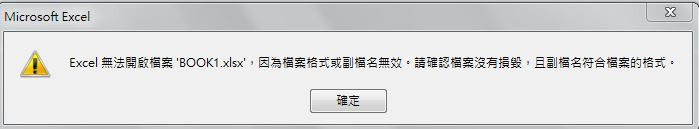froce iT邦大師 1 級 ‧ 2019-07-03 08:32:03 檢舉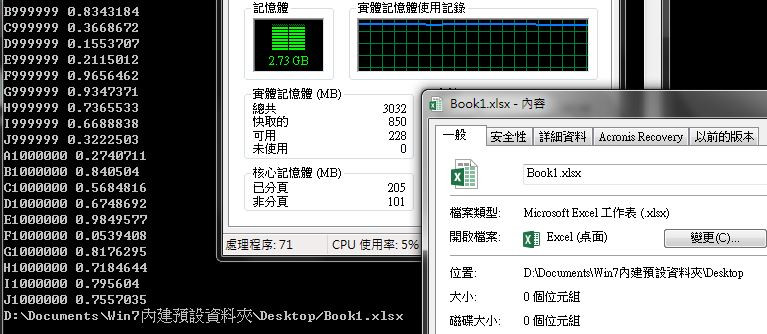froce有結果了！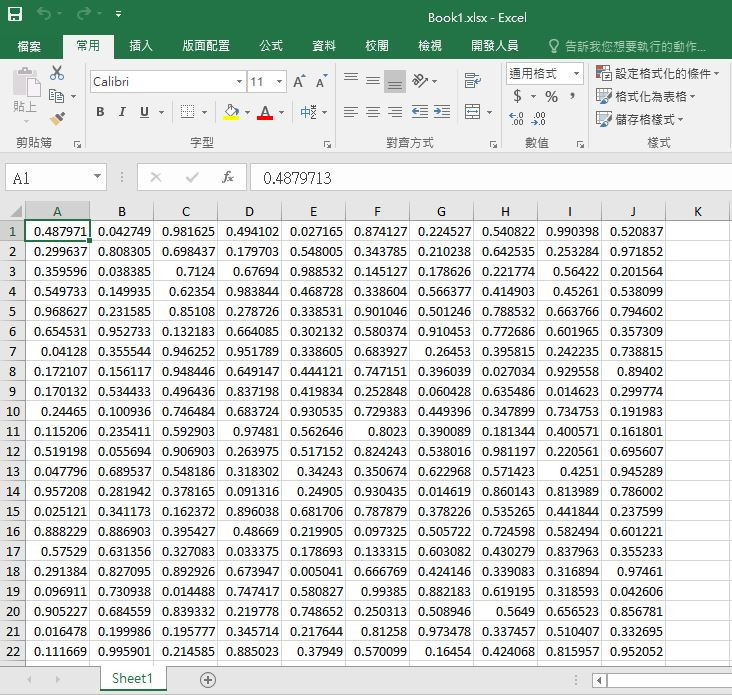froce iT邦大師 1 級 ‧ 2019-07-03 11:49:13 檢舉

froce 若您空檔時間有餘裕，也願意幫忙，改寫成 CSV版能減輕執行運算的硬體需求，當然再好不過了，感謝勞力！

froce iT邦大師 1 級 ‧ 2019-07-03 18:10:38 檢舉
``````package main

import (
"encoding/csv"
. "fmt"
"math/rand"
"os"
"path"
"path/filepath"
"strconv"
"time"
)

func main() {
start := time.Now().UnixNano()
ex, err1 := os.Executable()
if err1 != nil {
panic(err1)
}

exPath := filepath.Dir(ex)
sheet := path.Join(exPath, "Book1.csv")
csvFile, err2 := os.Create(sheet)
if err2 != nil {
panic(err2)
}
defer csvFile.Close()

csvWriter := csv.NewWriter(csvFile)

rand.Seed(time.Now().UnixNano())
p := rand.Perm(10000000)
rp := [][]string{}

for i := 0; i < 1000000; i++ {
_p := make([]string, 10, 10)

for index, elm := range p[i*10 : (i+1)*10] {
value := strconv.FormatFloat(float64(elm)/float64(10000000), 'f', -1, 64)
_p[index] = value
}

rp = append(rp, _p)

}

err3 := csvWriter.WriteAll(rp)
if err3 != nil {
panic(err3)
} else {
csvWriter.Flush()
}
end := time.Now().UnixNano()
Println("花費時間：", float64(end-start)/float64(1000000000), "秒")
}

``````

https://drive.google.com/file/d/1as9vj6j3d-T4qL4B__CoP5WzVmgof6Og/view?usp=sharing

CSV版記憶體佔用不到1G...

froce iT邦大師 1 級 ‧ 2019-07-03 18:42:05 檢舉

python：

``````import csv
import random as rn
import time

start = time.time()

_ = [str(i/10000000) for i in range(10000000)]
rn.shuffle(_)

with open("./test.csv", "w", encoding="utf8") as csvfile:
writer = csv.writer(csvfile)

for i in range(1000000):
writer.writerow(_[i*10:(i+1)*10])

end = time.time()
print("花費時間：", end-start, "秒")
``````

froce 我滿懷感激地收下 randomToCSV.exe檔案，感謝大力相助！froce iT邦大師 1 級 ‧ 2019-07-04 08:18:35 檢舉

GO得一直轉型，限制也多，重點是沒有map、filter這類的語意式函式，讓歷遍只能寫迴圈去做。

1

1、不考慮亂數重覆的前題，以下程式產生不到2分鐘
(i5-3470處理器、8GB DDR3，SSD、Windows10)

``````using OfficeOpenXml; //EPPLUS
using System;
using System.Diagnostics;
using System.IO;

namespace bigRandom
{
class Program
{
static void Main(string[] args)
{
if (File.Exists("export.xlsx")) File.Delete("export.xlsx");
ExcelPackage excel = new ExcelPackage(new System.IO.FileInfo("export.xlsx"));
ExcelWorksheet ews = excel.Workbook.Worksheets.Add("輸出結果");

int QRow = 1;
int QCol = 1;
int xx = 1;
for(int i = 0;i<10000000;i++)
{
Random Rng = new Random(Guid.NewGuid().GetHashCode());
int x = Rng.Next(0, 1000000000); //產生亂數
ews.Cells[QRow, QCol].Value = (double)x / 1000000000;
QCol++; //從A跑到J
if (QCol > 10) //如果下一行超過 J
{
QCol = 1;//回到A
QRow++;  //換行
}
}
excel.Save();
if (File.Exists("export.xlsx")) Process.Start("export.xlsx");
}
}
}

``````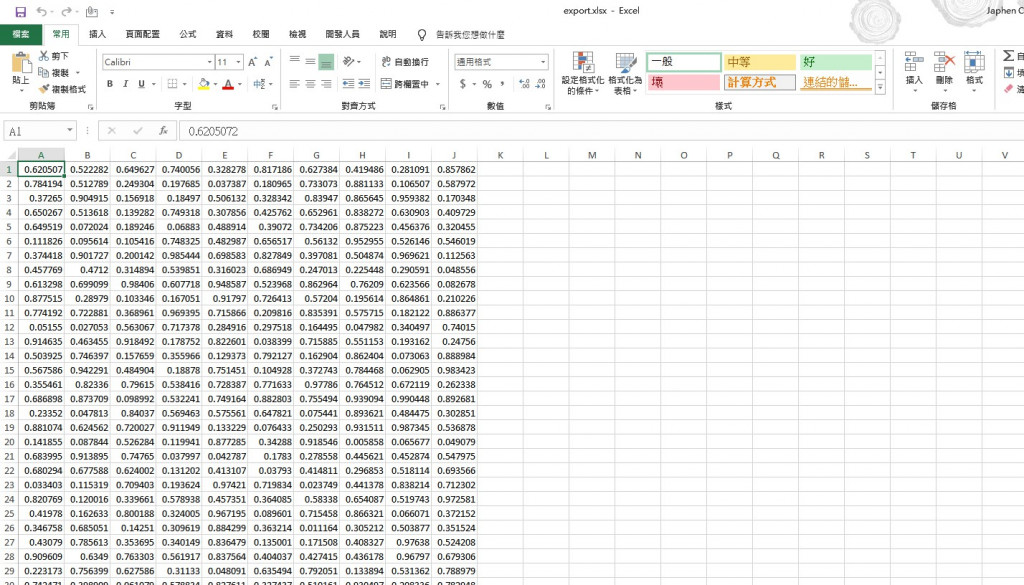2、考慮不可重覆.................

``````using OfficeOpenXml;
using System;
using System.Collections.Generic;
using System.Diagnostics;
using System.IO;

namespace bigRandom
{
class Program
{
static void Main(string[] args)
{
if (File.Exists("export.xlsx")) File.Delete("export.xlsx");
ExcelPackage excel = new ExcelPackage(new System.IO.FileInfo("export.xlsx"));
ExcelWorksheet ews = excel.Workbook.Worksheets.Add("輸出結果");

List<int> ballbag = new List<int>();// 球袋
for (int b = 1; b < 10000000; b++)
ballbag.Add(b); //產生1000萬個號碼球並放入球袋裡

int QRow = 1;
int QCol = 1;
while (ballbag.Count > 0) // 一直跑到球袋抽空為止
{
Random Rng = new Random(Guid.NewGuid().GetHashCode());
int x = Rng.Next(0,ballbag.Count); //從袋中取出號碼球
ews.Cells[QRow, QCol].Value = (double)ballbag[x] / 100000000; // 抽出的球所代表的數值除以1000萬01
ballbag.RemoveAt(x);
QCol++; //從A跑到J
if (QCol > 10) //如果下一行超過J，則換行
{
QCol = 1; //
QRow++;
}
}
excel.Save();
if (File.Exists("export.xlsx")) Process.Start("export.xlsx");
}
}
}

``````
froce iT邦大師 1 級 ‧ 2019-07-03 14:45:24 檢舉

python慢是一定的，只是沒想到C#也要弄到3小時...

froce iT邦大師 1 級 ‧ 2019-07-04 11:33:43 檢舉

C＃沒有直接對list做shuffle的函式嗎？

``````private static Random rng = new Random();

public static void Shuffle<T>(this IList<T> list)
{
int n = list.Count;
while (n > 1) {
n--;
int k = rng.Next(n + 1);
T value = list[k];
list[k] = list[n];
list[n] = value;
}
}

//用法:

List<Product> products = GetProducts();
products.Shuffle();
``````
0

1.所以我用如下的先各自生成100萬筆的數據10組。每組的數值範圍不同。

2.再產生資料前會先亂數處理後再儲存。

``````for (\$i = 1; \$i <= 10000000; \$i++) {
\$a[] = \$i;
}
shuffle(\$a);
``````